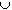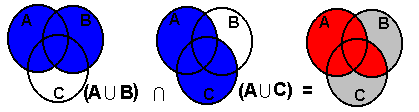#Interactive Real Analysis

Next | Previous | Glossary | Map

## 1.1. Notation and Set Theory

### Proposition 1.1.3: Distributive Law for Sets

A(BC) = (AB)(AC)

A(BC) = (AB)(AC)

### Proof:

These relations could be best illustrated by means of a Venn Diagram.Venn Diagram illustrating A(BC)Venn Diagram for (AB)(AC)

Obviously, the two resulting sets are the same, hence proving' the first law. However, this is not a rigorous proof, and is therefore not acceptable. Here is a real' proof of the first distribution law:

If x is in A union (B intersect C) then x is either in A or in (B and C). Therefore, we have to consider two cases:

• If x is in A, then x is also in (A union B) as well as in (A union C). Therefore, x is in (A union B) intersect (A union C).
• If x is in (B and C), then x is in (A union B) because x is in B, and x is also in (A union C), because x is in C. Hence, again x is in (A union B) intersect (A union C). This proves that
• A(BC)(AB)(AC)
To finish the proof, we have to prove the reverse inequality. So, take x in (A union B) intersect (A union C). Then x is in (A or B) as well as in (A or C).
• If x is in A, then x is also in A union (B intersect C).
• If x is in B, then it must also be in C. Hence, x is in B intersect C, and therefore it is in A union (B intersect C). That shows that
• A(BC)(AB)(AC)
Both inequalities together prove equality of the two sets.

The second distributive laws can be proved the same way, and is left as an exercise.Next | Previous | Glossary | Map